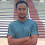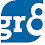# Averages - Examples

### Sol :             Average Income of 3 Friends = Rs. 6,600             So, Total Income of 3 friends = 3 X 6,600 = Rs. 19,800             Average income of 2 friends = 2 X 6,500             So, Total income of 2 friends = 2 X 6,500 = 13,000             Total income of 5 friends = Rs. 19,800 + Rs. 13,000                                                                           = Rs. 32,800             Average income of 6 friends = Rs.6,400             Total income of 6 friends = 6 x 6,400 = Rs. 38,400        So, income of 6th friend = Total income of 6 friends - Total income of 5 friends                                               = Rs.38,400 - Rs.32,800 = Rs.5,600 The average of Ramesh's marks in English, Maths and Science is 75 and the average of his marks in English, Maths, Science and Hindi is 70. How many marks did he score in Hindi? Sol :             The average marks in English, maths and Science is 75                   So, Total marks in English, maths and Science are 3 X 75 = 225             The average marks in English, Maths, Science and Hindi are 70.                   So, Total marks in English, Maths, Science and Hindi are 4 X 70 = 280                       So, Marks in Hindi = 280 - 225 = 55    The average age of the husband, his Wife and their Son ten years ago was 25 Years and that of the husband and wife six years ago was 37 years. What is the present age of the son (in years) ? Sol :           Average age of the husband, his wife and their son ten years ago was 25 years            Total of present ages of husband, wife and their son                                                         = (25 x 3) + 10 + 10 + 10 = 105 Years           Average age of the husband and his wife six years ago was 37 years           Total of present ages of husband and wife                                                                       = (37 X 2) + 6 + 6 = 86           So, Son's present age = 105 - 86 = 19 Years The average of A and B is 30 years. If C were to replace A, the average age would be 40 years and if he were to replace B, the average age would be 35 years. What is A's age? Sol :            Average age of A and B = 30 Years            Total age of A and B = 30 x 2 = 60 years ---------- (1)           Average age of B and C = 40 Years             Total age of B and C = 40 X 2 = 80 years ---------- (2)            Average age of A and C = 35 years               Total age of A and C = 35 X 2 = 70 years ---------- (3)             After adding (1) and (3) , we get                        2A + B + C = 130                   But, we know that (B + C) = 80                            So, 2A + B + C = 130                                                     => A = (130 - 80) / 2 = 25                      So, A's age is 25 years.Thats all for now friends... Tomorrow we shall do some more practice problems on this... Share with your friends if you like this post... Happy Reading :)

1.very good job by you people....really helpful to us....thanks a lot ....

2.superb .....thank you very much.

3.its a good job ........its really helpful to us........thnks to u...

4.but not enough for PO aspirants
plz provide some high level of questions
most importantly with shotrcut tricks
thank's for yr gr8 work
god bless u all
thank's once again

1.Agreed Friend. We surely will try to increase the difficulty level in our upcoming posts. Good Day :)

5.thnx...and ur website is very much useful.....it wil be really more helpful if
u upload some practice sets like mock exam

6.superb its really a short method to wrk out hatsoff please include some more prblms

mani

7.want more questions pls.......

8.Its superb...:-) But there is no shorcut..its lengthy.try to do something 4 that... Thank u

9.10.Its good. but i need shortcut method.

11.thanks very useful

12.13.PL. PROVIDE MORE DIFFICULT QUESTION AND ANSWERS

14.thanks ...hope it ll help...

15.Thanks............

16.very useful thanks :)

17.